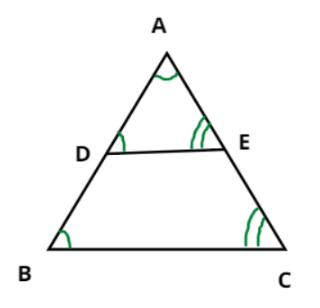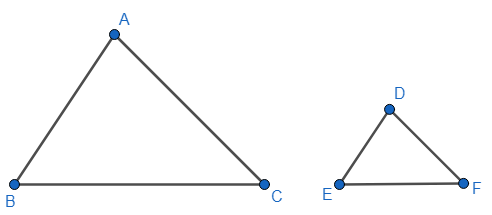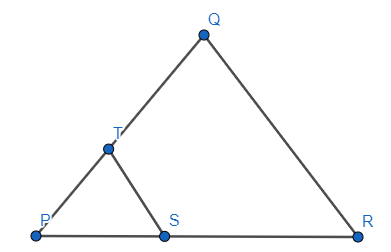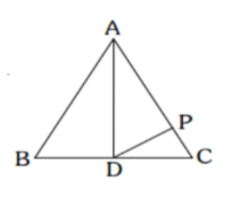Filters
Latest Questions
CBSE
Mathematics
Areas of similar triangles
Prove that the ratio of the areas of two similar triangles is equal to the ratio of the square of their corresponding sides.
CBSE
Mathematics
Areas of similar triangles
In a triangle ABC, DE is parallel to BC. If $\dfrac{{AD}}{{DB}} = \dfrac{2}{3}$ and AC = 18 cm, then find AE.
CBSE
Mathematics
Areas of similar triangles
In the given figure, DE is parallel to BC
${\text{(i)}}$ If ${\text{DE = 4 cm, BC = 6 cm}}$ and ${\text{Area(}}\vartriangle {\text{ADE) = 16 c}}{{\text{m}}^{\text{2}}}$, find the area of $\vartriangle {\text{ABC}}$.
${\text{(ii)}}$ If ${\text{DE = 4 cm, BC = 8 cm}}$ and ${\text{Area(}}\vartriangle {\text{ADE) = 25 c}}{{\text{m}}^{\text{2}}}$, find the area of $\vartriangle {\text{ABC}}$.
${\text{(iii)}}$ If ${\text{DE:BC = 3:5}}$. Calculate the ratio of the areas of $\vartriangle {\text{ADE}}$ and the area of BCED.CBSE
Mathematics
Areas of similar triangles
If the areas of two similar triangles are equal, prove that they are congruent.

CBSE
Mathematics
Areas of similar triangles
If $\Delta ABC\sim \Delta PQR,\dfrac{ar\left( \Delta ABC \right)}{ar\left( \Delta PQR \right)}=\dfrac{9}{4}$ and $PQ=8$ , then find the length of AB.
CBSE
Mathematics
Areas of similar triangles
Puneet prepared two posters on ‘National Integration’ for decoration on Independence Day on triangular sheets (say ABC and DEF). The sides AB and AC and the perimeter ${{P}_{1}}$ of $\Delta ABC$ are respectively three times the corresponding sides DE and DF and the perimeter ${{P}_{2}}$ of $\Delta DEF$. Are the two triangular sheets similar? If yes, find $\dfrac{ar\left( \Delta ABC \right)}{ar\left( \Delta DEF \right)}$.
What values can be inculcated through celebration of national festivals?CBSE
Mathematics
Areas of similar triangles
In the given figure, $ST||RQ$, $PS=3$ cm and $SR=4$ cm. find the ratio of the area of $\Delta PST$ to the area of $\Delta PRQ$.CBSE
Mathematics
Areas of similar triangles
The corresponding altitudes of two similar triangles are 3 cm and 5 cm respectively. Find the ratio between their areas.

CBSE
Mathematics
Areas of similar triangles
If $\Delta ABC \sim DEF$ , $AB:DE = 3:4$ , then find the ratio of area of triangles.

CBSE
Mathematics
Areas of similar triangles
AD is the median of $\Delta ABC$ and P is a point on AC such that
Area of $\Delta ADP$ : Area of $\Delta ABD$ = 2 : 3 and Area of $\Delta PCD$ : Area of $\Delta ABC$ = 1 : a. Find the value of a.CBSE
Mathematics
Areas of similar triangles
In a triangle ABC with $\angle BCA = 90^\circ$, the perpendicular bisector of AB intersects segments AB and AC at X and Y , respectively. If the ratio of the area of quadrilateral BXYC to the area of triangle ABC is $13:18$ and $BC = 12$, then what is the length of AC?

CBSE
Mathematics
Areas of similar triangles
The base and height of $\Delta LMN$ are 8cm and 6cm respectively. The base and height of $\Delta DEF$ are 10cm and 4cm respectively. Write the ratio $A\left( \Delta LMN \right):A\left( \Delta DEF \right)$ .
Prev
1
2
3